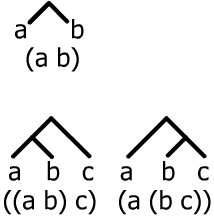#### You may also like### Doodles

Draw a 'doodle' - a closed intersecting curve drawn without taking pencil from paper. What can you prove about the intersections?### Russian Cubes

I want some cubes painted with three blue faces and three red faces. How many different cubes can be painted like that?### N000ughty Thoughts

How many noughts are at the end of these giant numbers?

# Counting Binary Ops

##### Age 14 to 16Challenge Level

This question is about the number of ways of combining an ordered list of terms by repeating a single binary operation.

For example with three terms $a$, $b$ and $c$ there are just two ways $((a\oplus b)\oplus c)$ and $(a\oplus (b\oplus c))$. Suppose the binary operation $\oplus$ is just ordinary subtraction and $a=12, \; b=7, \; c=5$ then $((a\oplus b)\oplus c)= 5 - 5 =0$ and $(a\oplus (b\oplus c))= 12 - 2 = 10$.

We are not concerned in this question with doing the 'arithmetic' or with whether the answers are the same or different. We just want to find out how many ways there are of combining the terms, or if you like of putting brackets into the expression. Note that we need the brackets because the answers may be different as in the subtraction example.

These two tree diagrams show the two cases for combining 3 terms."Show that for four terms, (three binary operations) there are five cases and find the number of cases for five terms and six terms.